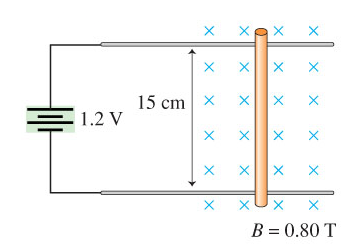# Problem: A device called a railgun uses the magnetic force on currents to launch projectiles at very high speeds. An idealized model of a railgun is illustrated in the figure. A 1.2 V power supply is connected to two conducting rails. A segment of copper wire, in a region of uniform magnetic field, slides freely on the rails. The wire has a 0.61 mΩ resistance and a mass of 9.0 g. Ignore the resistance of the rails. At some moment, the power supply is switched on. What will be the wire's speed after it has slid a distance of 1.0 mm?

###### FREE Expert Solution

Acceleration of the wire from Newton's second law:

$\overline{){\mathbf{a}}{\mathbf{=}}\frac{\mathbf{F}}{\mathbf{m}}}$, where F is force and m is the mass of the wire.

The force, F:

$\overline{){\mathbf{F}}{\mathbf{=}}{\mathbf{B}}{\mathbf{i}}{\mathbf{L}}}$

Let's also consider the kinematic equation:

80% (220 ratings)###### Problem Details

A device called a railgun uses the magnetic force on currents to launch projectiles at very high speeds. An idealized model of a railgun is illustrated in the figure. A 1.2 V power supply is connected to two conducting rails. A segment of copper wire, in a region of uniform magnetic field, slides freely on the rails. The wire has a 0.61 mΩ resistance and a mass of 9.0 g. Ignore the resistance of the rails. At some moment, the power supply is switched on. What will be the wire's speed after it has slid a distance of 1.0 mm?